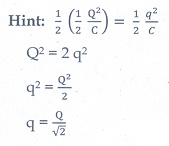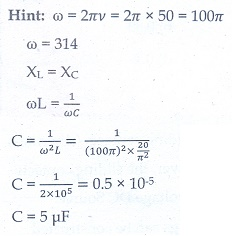Home | | Physics 12th Std | Electromagnetic Induction and Alternating Current: Multiple Choice Questions

# Electromagnetic Induction and Alternating Current: Multiple Choice Questions

Physics : Electromagnetic Induction and Alternating Current: Multiple choice questions with answers / choose the correct answer with answers - Physics Book back 1 mark questions and answers with solution

Multiple Choice Questions

1. An electron moves on a straight line path XY as shown in the figure. The coil abcd is adjacent to the path of the electron. What will be the direction of current, if any, induced in the coil?(a)ŌĆé The current will reverse its direction as the electron goes past the coil

(b)ŌĆé No current will be induced

(c)ŌĆé abcd2. A thin semi-circular conducting ring (PQR) of radius r is falling with its plane vertical in a horizontal magnetic field B, as shown in the figure.The potential difference developed across the ring when its speed v , is

(a) Zero

(b)  BvŽĆr2 /2 and P is at higher potential

(c) ŽĆrBv and R is at higher potential

(d) 2rBv and R is at higher potential3.The flux linked with a coil at any instant t is given by ╬”B = 10t 2 ŌłÆ 50t + 250. The induced emf at t = 3s is

(a) ŌłÆ190 V

(b) ŌłÆ10 V

(c) 10 V

(d) 190 V4.When the current changes from +2A to ŌłÆ2A in 0.05 s, an emf of 8 V is induced in a coil. The co-efficient of self-induction of the coil is

(a) 0.2 H

(b) 0.4 H

(c) 0.8 H

(d) 0.1 H5.The current i flowing in a coil varies with time as shown in the figure. The variation of induced emf with time would beAns: (a)6.A circular coil with a cross-sectional area of 4 cm2 has 10 turns. It is placed at the centre of a long solenoid that has 15 turns/cm and a cross-sectional area of 10 cm2. The axis of the coil coincides with the axis of the solenoid. What is their mutual inductance?

(a) 7.54 ┬ĄH

(b)  8.54 ┬ĄH

(c)  9.54 ┬ĄH

(d)  10.54 ┬ĄH7. In a transformer, the number of turns in the primary and the secondary are 410 and 1230 respectively. If the current in primary is 6A, then that in the secondary coil is

(a)  2 A

(b)  18 A

(c)  12 A

(d)  1 A8. A step-down transformer reduces the supply voltage from 220 V to 11 V and increase the current from 6 A to 100 A. Then its efficiency is

(a)  1.2

(b)  0.83

(c)  0.12

(d)  0.99. In an electrical circuit, R, L, C and AC voltage source are all connected in series. When L is removed from the circuit, the phase difference between the voltage and current in the circuit is ŽĆ /3 . Instead, if C is removed from the circuit, the phase difference is again ŽĆ / 3 . The power factor of the circuit is

a)     1 / 2

b)    1/ ŌłÜ2

c)     1

d)    ŌłÜ3 / 210.In a series RL circuit, the resistance and inductive reactance are the same. Then the phase difference between the voltage and current in the circuit is

a)    ŽĆ/4

b)    ŽĆ/2

c)     ŽĆ/6

d)    zero11.In a series resonant RLC circuit, the voltage across 100 Ōä” resistor is 40 V. The resonant frequency Žē is 250 rad/s. If the value of C is 4 ┬ĄF, then the voltage across L is

(a)  600 V

(b)  4000 V

(c)  400V

(d)  1 V12. An inductor 20 mH, a capacitor 50 ╬╝F and a resistor 40ŌĆå╬® are connected in series across a source of emf v = 10 sin 340 t. The power loss in AC circuit is

(a) 0.76 W

(b) 0.89 W

(c) 0.46 W

(d) 0.67 W13.  The instantaneous values of alternating current and voltage in a circuit areThe average power in watts consumed in the circuit is

a)     1 / 4

b)    ŌłÜ3 / 4

c)     1 / 2

d)    1/814. In  an  oscillating LC  circuit,  the maximum charge on the capacitor is Q. The charge on the capacitor when the energy is stored equally between the electric and magnetic fields is

a)     Q/2

b)    Q / ŌłÜ3

c)     Q /ŌłÜ2

d)    Q15. 20/ŽĆ2 H  inductor is connected to a capacitor of capacitance C. The value of C in order to impart maximum power at 50 Hz is

(a) 50 ╬╝F

(b) 0.5 ╬╝F

(c) 500 ╬╝F

(d) 5 ╬╝FTags : Physics , 12th Physics : Electromagnetic Induction and Alternating Current
Study Material, Lecturing Notes, Assignment, Reference, Wiki description explanation, brief detail
12th Physics : Electromagnetic Induction and Alternating Current : Electromagnetic Induction and Alternating Current: Multiple Choice Questions | Physics